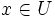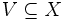# Locally Euclidean space

This article defines a property of topological spaces: a property that can be evaluated to true/false for any topological space|View a complete list of properties of topological spaces

Some people use the term non-Hausdorff manifold for locally Euclidean spaces that are not manifolds; however, by the convention on this wiki, Hausdorffness is part of the condition for manifolds. Learn more at convention:Hausdorffness assumption

## Definition

### Locally Euclidean of a fixed dimension

A topological space$X$ is termed locally$m$-Euclidean for a nonnegative integer$m$ such that it satisfies the following equivalent conditions:

1. For any point$x \in X$, there exists an open subset$U \subseteq X$ such that$x \in U$, and$U$ is homeomorphic to the Euclidean space$\R^m$.
2. For any point$x \in X$, there exists an open subset$U \subseteq X$ such that$x \in U$, and$U$ is homeomorphic to an open subset of Euclidean space$\R^m$.
3. For any point$x \in X$, and any open subset$V \subseteq X$, there exists an open subset$U$ of$X$ such that$x \in U \subseteq V$, and$U$ is homeomorphic to Euclidean space$\R^m$>

The equivalence of the three definitions follows from the fact that any Euclidean space is self-based: it has a basis of open subsets all of which are homeomorphic to the whole space.

### Locally Euclidean of possibly varying dimension

The term locally Euclidean is also sometimes used in the case where we allow the$m$ to vary with the point. In other words, the equivalent conditions (1)-(3) must hold, but the nonnegative integer could vary with the point.

This case could arise if the space has multiple connected components that have different dimensions. For instance, a disjoint union of a plane and a line is locally 2-Euclidean at the points on the plane and locally Euclidean at the points on the line.

## Formalisms

### In terms of the locally operator

This property is obtained by applying the locally operator to the property: Euclidean space

The equivalence between multiple sense of the term "locally" follows from the fact that any Euclidean space is self-based.

## Relation with other properties

### Stronger properties

Property Meaning Proof of implication Proof of strictness (reverse implication failure) Intermediate notions
manifold We assume additionally the conditions of Hausdorff and second-countable. We also require the dimension to be the same at all points. (obvious) The line with two origins is an example of a locally Euclidean space which is not a manifold, and also shows how many properties that we prove for manifolds, fail to hold for arbitrary locally Euclidean spaces. |FULL LIST, MORE INFO

### Weaker properties

Property Meaning Proof of implication Proof of strictness (reverse implication failure) Intermediate notions
locally contractible space has a basis of contractible spaces follows from the fact that Euclidean implies contractible a pair of intersecting lines is locally contractible but not locally Euclidean (at the point of intersection) |FULL LIST, MORE INFO
locally path-connected space has a basis of path-connected spaces (via locally contractible) (via locally contractible) Locally contractible space|FULL LIST, MORE INFO
locally metrizable space every point is contained in a metrizable open subset follows from the fact that Euclidean implies metrizable, using the Euclidean metric the pair of intersecting lines is metrizable (and hence locally metrizable) but not locally Eucldiean |FULL LIST, MORE INFO
locally normal space has a basis of normal spaces (via locally metrizable) (via locally metrizable) |FULL LIST, MORE INFO
locally Hausdorff space every point is contained in a Hausdorff open subset (via locally metrizable) (via locally metrizable) |FULL LIST, MORE INFO

### Manifold properties not satisfied for locally Euclidean spaces

Example Property failed Hausdorff? Second-countable? Dimension Other intermediate properties (that are always satisfied by manifold) that it satisfies
line with two origins Hausdorff space No Yes 1
Prufer manifold normal space Yes No 2
long line metrizable space Yes No 1 normal space
dictionary plane manifold Yes No 1 metrizable space, normal space